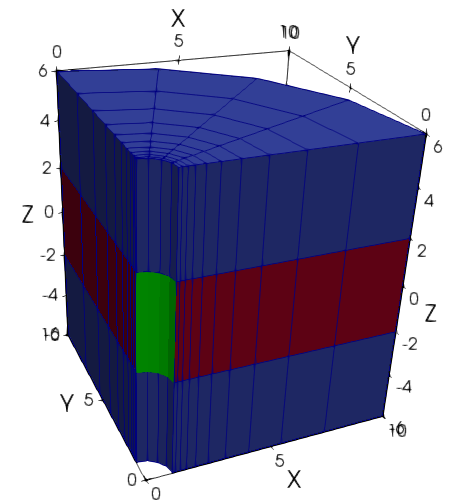# Contents

• Page 01 A single fluid

• Page 02 Numerical issues

• Page 03 Adding heat advection and conduction

• Page 04 Adding solid mechanics

• Page 05 Using a realistic equation of state for the fluid

• Page 06 Adding a tracer

• Page 07 A chemically reactive tracer with porosity and permeability changes

• Page 08 The PorousFlowSink and unsaturated flow

• Page 09 An overview of the PorousFlow architecture

• Page 10 Unleashing the full power of PorousFlow: using Kernels and Materials

• Page 11 Two-phase THM borehole injection

• Page 12 Boundary sinks and sources, and polyline sinks and sources (boreholes and rivers)

• Page 13 More elaborate chemistry

# Introduction

The PorousFlow module is a library of physics for fluid and heat flow in porous media. It is formulated in a general manner, so is capable of solving problems with an arbitrary number of phases, fluid components and chemical reactants. This tutorial guides the user through some commonly-used aspects of PorousFlow. Lots of core documentation may be found in Porous Flow.

This tutorial concerns fluid injection through a borehole into a large fluid-filled reservoir. The borehole is vertical, and cylindrical symmetry around the borehole axis is assumed. The tutorial begins with simple Darcy flow, and gradually adds more complex phenomena such as coupling with heat and solid mechanics, multi-phase flows and chemical reactions.

After conceptual modelling, the first step in any finite-element simulation is to create the mesh. The 3D mesh used for this tutorial is deliberately coarse (so that the tutorial input files may be easily run on the smallest computers) and is generated using MOOSE's inbuilt meshing capabilities. Firstly, a 2D annular mesh is created:

[Mesh]
type = AnnularMesh
dim = 2
nr = 10
rmin = 1.0
rmax = 10
growth_r = 1.4
nt = 4
tmin = 0
tmax = 1.57079632679
[]

(modules/porous_flow/examples/tutorial/00.i)

The radius of the borehole is 1m, the radius of the model is 10m, and only of the annulus is considered.

Now a sequence of MeshModifiers are applied to this 2D mesh. First, it is extruded to be 12m high using:

  [./make3D]
type = MeshExtruder
extrusion_vector = '0 0 12'
num_layers = 3
bottom_sideset = 'bottom'
top_sideset = 'top'
[../]

(modules/porous_flow/examples/tutorial/00.i)

Then it is shifted downwards (ie, in the negative direction) by 6m to place the injection region around the origin:

  [./shift_down]
type = Transform
transform = TRANSLATE
vector_value = '0 0 -6'
depends_on = make3D
[../]

(modules/porous_flow/examples/tutorial/00.i)

An aquifer region is created in the central 6m:

  [./aquifer]
type = SubdomainBoundingBox
block_id = 1
bottom_left = '0 0 -2'
top_right = '10 10 2'
depends_on = shift_down
[../]

(modules/porous_flow/examples/tutorial/00.i)

and an injection area is created on the borehole's wall in the aquifer region:

  [./injection_area]
combinatorial_geometry = 'x*x+y*y<1.01'
included_subdomain_ids = 1
new_sideset_name = 'injection_area'
depends_on = 'aquifer'
[../]

(modules/porous_flow/examples/tutorial/00.i)

Finally, the subdomains are named "caps" (for the upper and lower caprock) and "aquifer":

  [./rename]
type = RenameBlock
old_block_id = '0 1'
new_block_name = 'caps aquifer'
depends_on = 'injection_area'
[../]

(modules/porous_flow/examples/tutorial/00.i)

This process has created the 3D mesh. Each of the input files in the tutorial follow this process. The result is shown in Figure 1.Figure 1: The 3D mesh. The aquifer is shown in red and the caprocks in blue. The green surface is the injection area.

If this were a real simulation rather than just a tutorial, it would be much more efficient to use cylindrical coordinates, which are called "RZ" coordinates in MOOSE. The mesh-generation process is

[Mesh]
type = GeneratedMesh
dim = 2
nx = 10
xmin = 1.0
xmax = 10
bias_x = 1.4
ny = 3
ymin = -6
ymax = 6
[]

(modules/porous_flow/examples/tutorial/00_2D.i)

with MeshModifiers:

[MeshModifiers]
[./aquifer]
type = SubdomainBoundingBox
block_id = 1
bottom_left = '0 -2 0'
top_right = '10 2 0'
[../]
[./injection_area]
combinatorial_geometry = 'x<1.0001'
included_subdomain_ids = 1
new_sideset_name = 'injection_area'
depends_on = 'aquifer'
[../]
[./rename]
type = RenameBlock
old_block_id = '0 1'
new_block_name = 'caps aquifer'
depends_on = 'injection_area'
[../]
[]

(modules/porous_flow/examples/tutorial/00_2D.i)